# Circle normal to a vector

• DivergentSpectrum

#### DivergentSpectrum

i have a 3d vector, and i want to draw a circle with a specified radius around the vector (for computer programming)
so i have the location of the center of the circle(first point of the vector)
but i also want the circle to be at a right angle to the vector

my approach: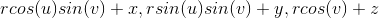the equation of a sphere centered at x,y,z.
but i need to find the relation between u and v so that it forms a circle normal to some specified vector

That might be possible, but it looks complicated.

I would find two vectors u,v orthogonal to the specified vector (let's call it "n") and orthogonal to each other. This can be done with the cross-product. Afterwards you can combine them with sin and cos as in the 2-dimensional case.

if a plane normal to a vector xn,yn,zn is given by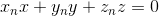then i think i could replace the parametric sphere coordinates with the coordinates to the plane and solve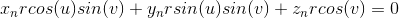what i want out of this is the relation between u and v, but i can't figure out how to solve it
then once i have u and v in terms of a single t parameter i can center it at the correct point.

do you mean the u,v vectors as in the parameters? or something else?

do you mean the u,v vectors as in the parameters?
Probably a bad choice of variable names in my post. I mean two vectors.
I don't like the sphere approach. It might be possible, but I don't see the solution there while I am sure the other method works.

To "draw a circle normal to a vector" the first thing you need to do is find a plane normal to the vector. That's easy- if the plane is given by $$A\vec{i}+ B\vec{j}+ C\vec{k}$$ then any plane normal to it is of the form $$Ax+ By+ Cz= D$$ for some constant D. Finding D is another matter. A vector does not have a specified position in space.

The center of the circle seems to be given as (x,y,z).

We don't know either because you did not show what you did.

Code:
            double theta = Math.Atan2(curly[i, j, k], curlx[i, j, k]);
double phi = Math.Acos(curlz[i, j, k] / Math.Sqrt(curlx[i, j, k] * curlx[i, j, k] + curly[i, j, k] * curly[i, j, k] + curlz[i, j, k] * curlz[i, j, k]));
double costheta = Math.Cos(theta);
double sintheta = Math.Sin(theta);
double cosphi = Math.Cos(phi);
double sinphi = Math.Sin(phi);

int l = 0;
double t ;
int nitert = 20;
double dt = 2 * Math.PI / nitert;
double[] xcircle = new double[nitert+1];
double[] ycircle = new double[nitert+1];
double[] zcircle = new double[nitert+1];
circlepoints.Add(new Point3D(-size * sinphi / 25 + xcurrent, size * cosphi / 25+ ycurrent, zcurrent));

while (l <= nitert)
{
t = l * dt;
xcircle[l] = -size * Math.Cos(t) * sinphi / 25 + size * Math.Sin(t) * costheta * cosphi / 25 + xcurrent;
ycircle[l] = size * Math.Cos(t) * cosphi / 25 + size * Math.Sin(t) * costheta * sinphi / 25 + ycurrent;
zcircle[l] = -size * Math.Sin(t) * sintheta / 25+ zcurrent;

l++;
}

I always feel bad asking people to look over my code lol.

anyway curlx,curly,curlz is the normal vector(yes I am doing a stokes theorem program :D)
and xcurrent,ycurrent,zcurrent is the position vector.

size/25 is the radius of the circle.

Hey, don't know if I understand completely but here is what I think (btw I am still getting used to using the latex math symbols here so I won't use them in this response)

Use the cross product (copied&pasted this one):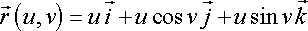Here the vector is normal to the circle's surface in the positive Z direction. That is the crossproduct of r's x component and y component.
Vector = rx cross ry

Continuation of my last post:

syms u v
>>R = [u 0 0; 0 u*cos(v) 0; 0 0 u*sin(v)]

R =

[ u, 0, 0]
[ 0, u*cos(v), 0]
[ 0, 0, u*sin(v)]

>> vector = cross(R(1,: ),R(2,: ) )

vector =

[ 0, 0, u^2*cos(v)]

*had to edit away the simily faces

Last edited:
the wolfram site says:
While a 2D circle is parameterized by only three numbers (two for the center and one for the radius), in 3D six are needed. One set of parametric equations for the circle in 2D is given by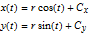and center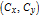.
In 3D, a parametric equation is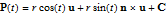,, center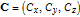, and normal vector(is the cross product). Here,is any unit vector perpendicular to. Since there are an infinite number of vectors perpendicular to, using a parametrizedis helpful. If the orientation is specified by a zenith angle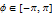and azimuth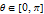, then,, and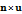can have simple forms: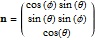,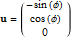,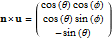.

but actually they made a mistake. here phi is the azimuth and theta is the zenith.
someone should probably tell themHey,

example similar to: http://tutorial.math.lamar.edu/Classes/CalcIII/ParametricSurfaces.aspx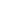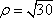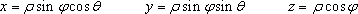Afterwards, its the same process I went thru above with the coneLast edited by a moderator: